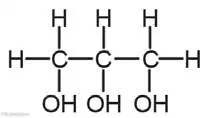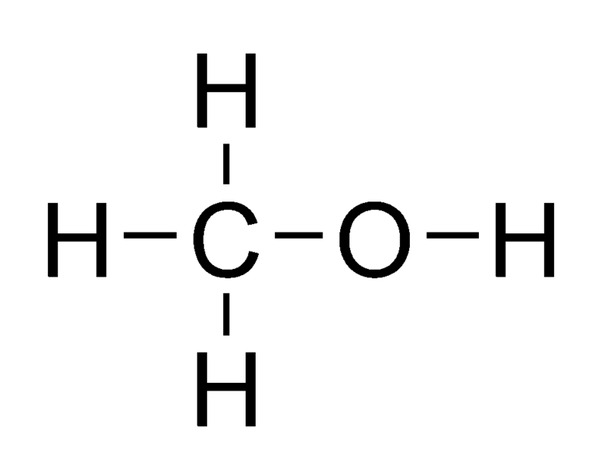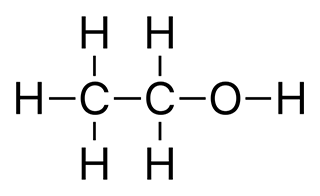# Hydrocarbon and its Compound

## 1 Marks Question

1. Write general formula of alkene.
2. General formula of alkene is CnH2n.
3. What is IUPAC name of methyl alcohol?
4. The IUPAC name of methyl alcohol is methanol.
5. What are acetylenes?
6. Hydrocarbons having three bonds between carbons are acetylenes.
7. What is the IUPAC name of ethyl alcohol?
8. The IUPAC name of methyl alcohol is ethanol.
9. Write two examples of saturated hydrocarbons.
10. Two examples of saturated hydrocarbons are methane, ethane.
11. Write the full form of IUPAC.
12. The full form of IUPAC is International Union of Pure and Applied Chemistry.
13. Write the molecular formula and structural formula of iso-butane.
14. The molecular formula of iso-butane is C4H10 and structural formula is shown below:15. Write the name of hydrocarbon used to make chloroform.
16. The name of hydrocarbon used to make chloroform is methane.
17. Write the structural formula and one use of glycerol.
18. Structural formula of glycerol is:It is used in the manufacture of good quality soaps and cosmetics.
19. Write name and structural formula of the alcohol used in sprit lamp.
20. Methyl alcohol is used in sprit lamp.
Its structural formula is:21. What types of hydrocarbons are called paraffins?
22. Saturated hydrocarbons are called paraffins.
23. What are isomers? Give an example of isomers.
24. Organic compounds with the same molecular formula but different structural formulas are isomers. For example, n-butane, iso-butane.
25. Write condensed formula and one use of glycerol.
26. Condensed formula of glycerol is C3H8O3.
It is used in the manufacture of good quality soaps and cosmetics.
27. Write name and one use of the given compound.28. It is methyl alcohol. It is used in making perfume.
29. Write full form of LPG.
30. The full form of LPG is Liquefied Petroleum Gas.
31. What is homologous series?
32. The series of organic compounds which are obtained from the same general formula, differ regularly by -CH2 group and have the same functional group is called homologous series.
33. Write the structural formula of ethyl alcohol.
34. The structural formula of ethyl alcohol is:35. Write the structural formula of glycerol.
36. The structural formula of glycerol is:37. Write the structural formula of trihydric alcohol.
38. The structural formula of trihydric alcohol is:39. Give the name of following compound.40. The name of given compound is ethene.
41. Write the name of compounds having the following structural formula.
H – C ≡ C – H
42. The name of compounds having the given structural formula is Acetylene (ethyne).
43. Which alcohol is used to manufacture formaldehyde?
44. Methyl alcohol is used to manufacture formaldehyde.